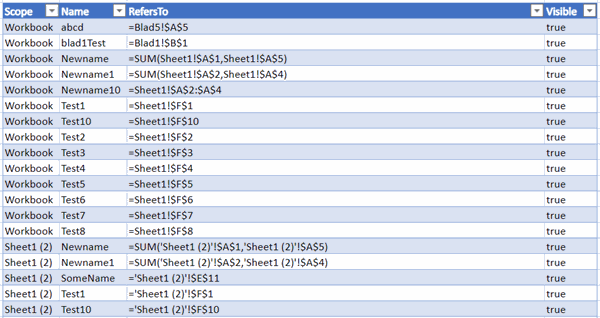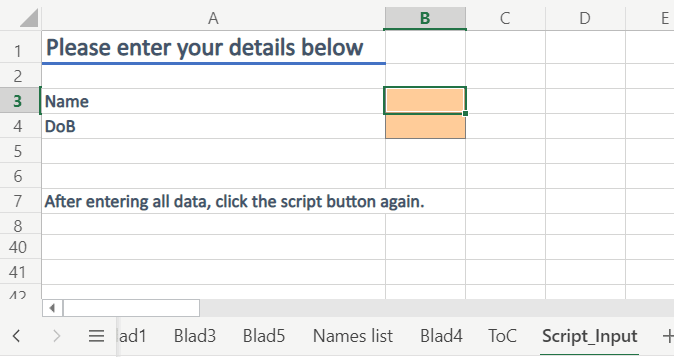Home > Article index > Office Script examples

# Excel Office Script Examples

## Introduction

Since July 2020, Microsoft 365 users can record their actions in Excel on-line into Office Script macros. Earlier, I've posted an article that shows you how to get started. On this page, I will list some example scripts which you cannot record but might come in handy.

Some of these scripts contain literal values, (the values between quotes). This means things like worksheet names are hard-coded into the example scripts: "ToC". So if you need a different worksheet name, edit the script accordingly. Because Microsoft has not yet given us a way to prompt the user for a value, we must edit the script to change what sheet it affects.

Working in Excel Online and need a table of Contents for your workbook quickly? Here's an Office Script that produces one. It generates a list for all worksheets and all charts, with hyperlinks pointing to their locations.

First let me show the main function and explain it step by step.

function main(workbook: ExcelScript.Workbook) {

if (workbook.getWorksheet("ToC")) {
let temp = workbook.getWorksheet("ToC")
temp.delete();
}

let allSheets = workbook.getWorksheets();
let reportCell = tocSheet.getRange("B2");
let newTable = addTocTable("Worksheets", workbook, reportCell);
reportCell = reportCell.getOffsetRange(20);

for (let i = 0; i < allSheets.length; i++) {
reportCell.getOffsetRange(i + 10).setValue(allSheets[i].getName());
}

reportCell = reportCell.getOffsetRange(newTable.getRowCount() + 20)
reportCell = reportCell.getOffsetRange(20)
let k = 0;
for (let i = 0; i < allSheets.length; i++) {
let allCharts = allSheets[i].getCharts();
for (let j = 0; j < allCharts.length; j++) {
k++;
let theSheet = allSheets[i];
reportCell.getOffsetRange(k, 0).setValue(allSheets[i].getName());
reportCell.getOffsetRange(k, 1).setValue(allCharts[j].getName());
let topLeftCell = getCellUnderChart(allCharts[j], allSheets[i]);
}
}
}

tocSheet.activate();
}

These are the important steps in this code:

• check whether a worksheet called ToC exists and if it does, delete it.
• Fetch a list of all worksheets and store that in a variable called allSheets
• Add a worksheet called "ToC" and assign it to a variable called tocSheet
• Assign cell B2 to the variable reportCell
• To ensure we start adding the sheet names on the first empty row of the table, we offset the reportCell with two so it now points to cell B4
• Now it is time to loop through the allSheets collection and list the name of each sheet in the first column of the table
• After looping through the sheets, we add the HYPERLINK formula to the second column, using the name of the worksheet in the first column to create a link to each worksheet
• We offset the reportCell position by adding the number of rows in the worksheet table (plus two rows extra for spacing)
• Now we add the heading for the charts table and an empty table to hold them, using that addTocTable function again
• The Charts table has four columns: Worksheet, Name, Location and Link
• We run through all worksheets and for every worksheet we
• run through all charts
• fill the chart table with the appropriate information: Worksheet, Name and Location (location required me to design a function called getCellUnderChart because Office Script does not have a TopLeftCell property of a chart like VBA has)
• There's a snag for charts on sheets which contain a space in their name; the correct address of the topleftCell in sheets like that have apostrophes around the name: 'Blad 2'!F1. However, if you write 'Blad 2'!F1 to a cell, Excel strips that first apostrophe. Hence the if statement that adds an additional apostrophe in such cases.
• Once we've done all charts on all worksheets, we place the HYPERLINK formula in the last column again using the address information in the third column

The main function uses two helper functions, here's the addTocTable one:

function addTocTable(caption: String, workbook: ExcelScript.Workbook, location: ExcelScript.Range): ExcelScript.Table {
location.setValue(caption);
location = location.getOffsetRange(20);
return newTable;
}

All it does is add the caption in the location cell, style it as "Heading 1" and add a new one-column table. The function returns the table as an object so we can use that object in our calling routine.

And here is the getCellUnderChart function:

function getCellUnderChart(cht: ExcelScript.Chart, ws: ExcelScript.Worksheet): ExcelScript.Range {
let topLeftCell = ws.getRange("A1");
let i = 0;
do {
i++;
topLeftCell = topLeftCell.getOffsetRange(0, i)
}
while (topLeftCell.getLeft() < cht.getLeft());
i = 0;
do {
i++;
topLeftCell = topLeftCell.getOffsetRange(i, 0)
}
while (topLeftCell.getTop() < cht.getTop());

return topLeftCell.getOffsetRange(-1, -1);
}

The basic principle is simple: the function first loops through the cells of the worksheet horizontally, starting from cell A1, until it has found a cell with a Left value that is greater than or equal to the chart's Left position. After that it does the same vertically to find the Top position. Because the two loops take us one column too far to the right and one row too far down, we offset the found cell by (-1, -1) so we return the correct cell which denotes where the top-left corner of the chart is located.

## Adding a list of Range names

Desktop Excel has a simple feature to add a list of range names to your workbook (Formulas tab, Use in Formula drop-down, Paste List). But Excel on-line does not (yet) have that feature. Here is an Office script which adds a worksheet calles "Names List" to your workbook, listing all range names and some of their properties.

function main(workbook: ExcelScript.Workbook) {
var RowCt = 1;
var sheetName = "";
let MyNames = workbook.getNames();
let selectedSheet = workbook.getWorksheet("Names list");
if (selectedSheet) {
selectedSheet.delete();
}
let worksheets = workbook.getWorksheets();
selectedSheet.getRange("B2:E2").setValues([["Scope""Name""RefersTo""Visible"]]);
for (let i = 0; i < MyNames.length; i++) {
RowCt++
selectedSheet.getCell(RowCt1).getResizedRange(03).setValues([
["Workbook"MyNames[i].getName(), "'" + MyNames[i].getFormula(), "'" + MyNames[i].getVisible()]]);
}
for (let i = 0; i < worksheets.length; i++) {
sheetName = worksheets[i].getName();
var myNames = worksheets[i].getNames();
for (let j = 0; j < myNames.length; j++) {
RowCt++;
selectedSheet.getCell(RowCt1).getResizedRange(03).setValues([
[sheetName, myNames[j].getName(), "'" + myNames[j].getFormula(), "'" + myNames[j].getVisible()]
]);
}
}
newTable.getRange().getFormat().autofitColumns();
}

The resulting table looks like this:## Prompting for user input

OfficeScript does not have a feature like the VBA InputBox, nor can you design input screens. So we need a work-around to get information from the user. The script below checks for presence of a worksheet called "Script_Input". If that sheet is not in the workbook, it gets added. Next a function is called which allows you to pass it a prompt and an array of inputs, which will be added to the Script_Input sheet. The resulting input sheet looks like this:If the script detects that the "Script_Input" sheet is available, it assumes all input has been completed and runs an action with that input (writes to the console).

This is the entire script:

function main(workbook: ExcelScript.Workbook) {
if (workbook.getWorksheet("Script_Input")) {
//we assume input is there, here are your script's main actions
let inputSheet = getInputSheet(workbook, "Script_Input");
let name = inputSheet.getRange("B3").getValue();
let dob = inputSheet.getRange("B4").getValue();
console.log(name + " " + dob);
}
else {
createPromptSheet(workbook, "Please enter your details below", ["Name""DoB"])
//It may be a good idea to delete the Script_Input sheet now
}
}

function createPromptSheet(workbook: ExcelScript.Workbook, prompt: string, questions: string[]) {
let inputSheet = getInputSheet(workbook, "Script_Input");
let titleCell = inputSheet.getRange("A1")
titleCell.setValue(prompt);
titleCell.getFormat().autofitColumns();
let questionCell = inputSheet.getRange("A3");
let rw = 0;
for (let i = 0; i < questions.length; i++) {
rw++;
questionCell.getOffsetRange(i, 0).setValue(questions[i]);
questionCell.getOffsetRange(i, 1).setPredefinedCellStyle("Input");
}
inputSheet.activate();
questionCell.getOffsetRange(rw + 20).setValue("After entering all data, click the script button again.");
questionCell.getOffsetRange(01).select();
}

function getInputSheet(workbook: ExcelScript.Workbook, name: string) {
if (workbook.getWorksheet(name)) {
return workbook.getWorksheet(name);
}
else {
}

}

Comment by: John from Ireland (24-8-2021 19:21:00) deeplink to this comment

This is an extremely useful script which I will use for my daily reporting needs.

Comment by: Jan Karel Pieterse (25-8-2021 11:00:00) deeplink to this comment

Hi John,

Thanks. Please note that I've updated the script today so it works correctly for both sheets with and without a space in the sheet name

Comment by: MIKE SMITH (27-8-2021 21:12:00) deeplink to this comment

Thanks for this. I have so many questions...
Does this code editor expand?
How do you debug?
Is there an equivalent of VBA's Immediate Window?

Comment by: Jan Karel Pieterse (27-8-2021 23:45:00) deeplink to this comment

Hi Mike,

We're limited to a maximum width of the editor.
Debugging is a struggle, there is no way to step the code for example so you have te revert to inserting console.log statements at strategic places. Cumbersome.
There is no immediate window.
But the intellisense is way better and indenting is automatic.

Comment by: LE GUEN Frédéric (29-8-2021 05:58:00) deeplink to this comment

Very good example to learn Office Script.
The way to select the target cells to write the result with getOffsetRange, getResizedRange is confusing for the moment for me. I have to practice ;)
Thanks for sharing this

Comment by: John from Ireland (14-12-2021 01:45:00) deeplink to this comment

There is work ongoing, I believe, to add the Office Scripts engine into Visual Studio. If you open an Office Scripts .ts file, in Visual Studio Code, it does not recognize the ExcelScript engine but there is a way of enabling it.
Here is the instructions for that:
https://github.com/sumurthy/officescripts-projects/tree/main/misc
You can edit the code there but not execute it. You have to copy-paste it back into the Excel Online Code Pane to do that.

The Excel Code editor pane, now, expands across the entire width of Excel.

Comment by: Jan Karel Pieterse (14-12-2021 09:58:00) deeplink to this comment

Thanks John, quite useful :-)

### Have a question, comment or suggestion? Then please use this form.

If your question is not directly related to this web page, but rather a more general "How do I do this" Excel question, then I advise you to ask your question here: www.eileenslounge.com.

To post VBA code in your comment, use [VB] tags, like this: [VB]Code goes here[/VB].